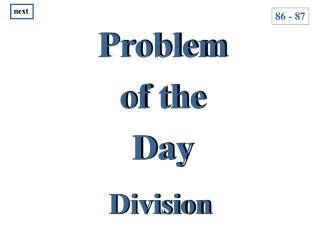Download PresentationProblem of the Day

# Problem of the Day - PowerPoint PPT PresentationDownload Presentation## Problem of the Day

- - - - - - - - - - - - - - - - - - - - - - - - - - - E N D - - - - - - - - - - - - - - - - - - - - - - - - - - -
##### Presentation Transcript

1. next 86 - 87 Problem of the Day Division

2. next Use the digits 1, 2, 3, and as many zeros as you want to form a division problem with a quotient of 70. 0 1 3 2

3. next Using the digits 1, 2, and 3, I must create a division problem that has 70 for a quotient. I know that I can use as many zeros as necessary. After looking at the digits, I can divide 21 by 3. My answer would be 7. In order to get 70, I would add a zero to 21 and get 210. When I divide 210 by 3, my answer would be 70.

4. next I could also add zeros to 210 and 3 to get 2100 ÷ 30. That would also give me 70. Thus, there are an infinite number of division problems that would give me 70 for a quotient. Each time I divided; my quotient would be 70.

5. next next Answers: 210 ÷ 3 2100 ÷ 30 21000 ÷ 300 7 0 3 3 3 2 1 2 1 0 2 1 0 0 0 0